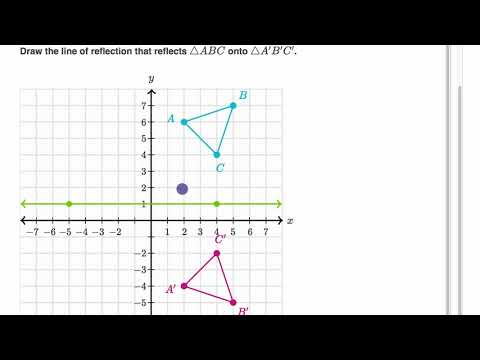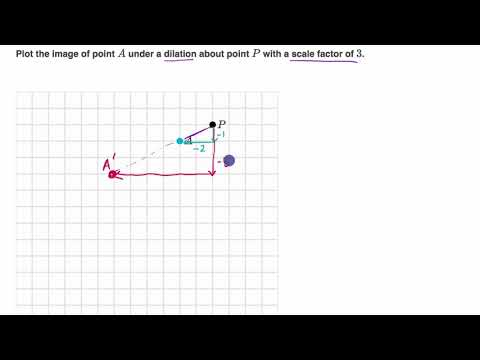Video

# Reflecting points (Full video)

Description: Reflecting points across horizontal and vertical lines, both graphically and algebraically. We're asked to plot the image of point A under a reflection across the line l. Well, one way to think about it is point A is exactly one, two, three, four units to the right of l. And so its reflection is going to be four units to the left of l. So here we're asked plot the image of point B under a reflection across the x-axis. Alright, so this is point B, and we're going to reflect it across the x-axis right over here. So if we were to reflect across the x-axis, essentially create its mirror image, it's going to be five units above the x-axis.

### Other videos you might be interested in### Determining reflections (Full video)